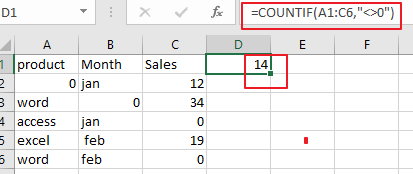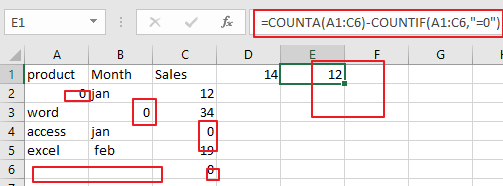# How to Count the Number of Cells with non-zero value in Excel

This post will guide you how to count the number of cells with non-zero value with formula in Excel 2013/2016.

## Count the Number of Cells with non-zero value

Assuming that you have a list of data in range A1:C6 which contain text string values. And you want to count the number of cells with non-zero value in your data. You can use a formula based on the COUNTIF function to accomplish the result. The formula is like below:

`=COUNTIF(A1:C6,"<>0")`

Type this formula into a blank cell and press Enter key on your keyboard, you would get the total number of non-zero values in the given range of cells.## Count the Number of Cells Ignoring Zeros and Blank Cells

The above formula can only be used to count the number of cells ignoring zero values, and if you also want to ignore blank cells at that same time while counting the cells in the given range of cell, you can use another formula based on the COUNTIF function and the COUNTA function. Just like below:

`=COUNTA(A1:C6)-COUNTIF(A1:C6,"=0")`

Type this formula into a blank cell and press Enter key on your keyboard.### Related Functions

• Excel COUNTIF function
The Excel COUNTIF function will count the number of cells in a range that meet a given criteria. This function can be used to count the different kinds of cells with number, date, text values, blank, non-blanks, or containing specific characters.etc.= COUNTIF (range, criteria)…
• Excel COUNTA function
The Excel COUNTA function counts the number of cells that are not empty in a range. The syntax of the COUNTA function is as below:= COUNTA(value1, [value2],…)…

### You might also like:

Sidebar